# Magnetic Susceptibility

Before defining the term magnetic susceptibility, we need to know some terms such as magnetic field (H), magnetic flux density (B) and magnetic permeability (µ)
Magnetic field : Lines of force will be produced which diffuses through the medium where the field is applied.
Magnetic flux density : The amount of lines of force per unit area.
Magnetic permeability : It denotes the relation between the magnetic field and magnetic flux density. (B = µH)

Now, Magnetic susceptibility can be simply defined as the measurement of the extent to which a substance can be magnetised by applying a peripheral magnetic field. It is denoted by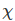(from the Greek letter chi). It can be measured as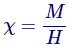M – magnetisation of the material
H – Applied peripheral magnetic field strength
Magnetic susceptibility is actually caused by the reciprocal action of electrons and nuclei by the application of peripheral magnetic field. That is, a c takes place inside it that will either opposes or intensify the applied peripheral field. If it opposes the applied magnetic field, the result will be diamagnetism.

If it intensifies the field (the electrons and nuclei which rotates in the same direction of magnetic field) it results in paramagnetic or ferromagnetic or super magnetism depending on the degree of intensification.
The range of the value of magnetic susceptibility () of different magnetic substances is

• Diamagnetic substances :< 0
• Paramagnetic substances : 1 >> 0
• Super paramagnetic and ferromagnetic substances :>> 1

Now, consider a material having magnetisation M which is placed under a peripheral magnetic field of B0 and the magnetic permeability of free space is µ0. Then, the total magnetic field in the material after applying peripheral magnetic field is given by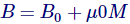When the internal magnetic field of the material is considered, µ0 can be substituted by µ which is given by µ = Km µ0. Where, Km is relative permeability. If the material does not produce any internal magnetism with applied magnetic field, then the value of Km will be equal to 1. When Km>1, due to the application of a peripheral magnetic field the material is magnetised. Thecan be related with Km, i.eis defined as how much value the relative permeability differs from one.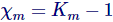m and Km will give same information. Both quantities are dimensionless.

### Magnetic Susceptibility and Temperature

The paramagnetic material will be in its magnetic state only when it is above a specific temperature. This particular temperature is called Curie temperature.
The relation of same with magnetic susceptibility is explained by Curie law which is given by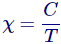C – Curie constant.
T – Temperature in Kelvin.
The paramagnetic material with weak interactive electrons has to obey the Curie-Weiss law which is given by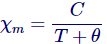m – Molar magnetic susceptibility
θ – Weiss constant
The Curie-Weiss plot can be obtained by plotting a graph of 1/m verses temperature. From that graph, we will get the curie constant by the inverting the slope and Weiss constant can be obtained from the y-intercept (Figure 1). If the Curie-Weiss law is satisfied then the plot should be linear.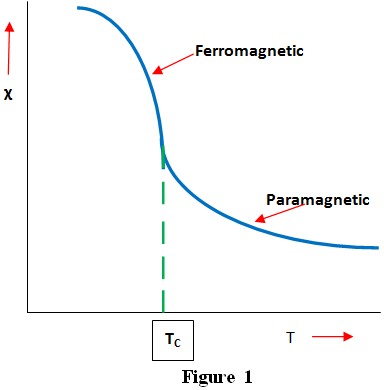### Magnetic Susceptibility Measurement

Basically, there is a sensor in an oscillator circuit. It produces a low energy alternating magnetic field. Any material which has magnetic susceptibility, when placed near the region of sensor, it will result in change in frequency of oscillator. The information of pulsed frequency is converted into magnetic susceptibility by the electronics. There are different methods used for the measurement of magnetic susceptibility. They are Faraday’s scale, Guoy’s scale, inductive method using squid magnetometer and MR method.

Want To Learn Faster? 🎓
Get electrical articles delivered to your inbox every week.
No credit card required—it’s 100% free.